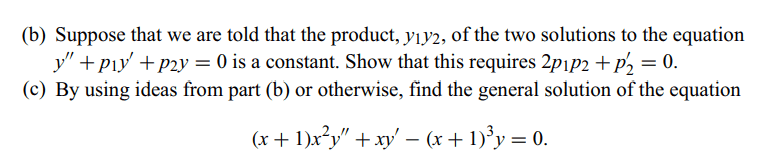# I need someone to help me solve this problem.

• MHB
Another1in problem b from $y_1y_2 = c$ so I was able to specify that $y_1 = c_1x^2$ abd $y_2 = c_2x^{-2}$
Correspond to $y_1y_2 = c_1c_2 = c = constant$ then I can find $y_1', y_1'', y_2',y_2''$
So. I can solve $2p_1p_2 +p_2' = 0$

But in problem C, I have no idea, so I assign $y = c_1x^r + c_2x^s$ but i can solve it

I select $y_1= c_1x^r$ and $y_2= c_2x^s$
so $(x+1)x^2y_1'' + xy_1' +(x+1)^3y_1 = 0$ and
$(x+1)x^2y_2'' + xy_2' +(x+1)^3y_2 = 0$ i find first and second deriative of y1 and y2
$r(r-1)(x+1) +rx - (x+1)^3 = 0$ $s(s-1)(x+1) +sx - (x+1)^3 = 0$